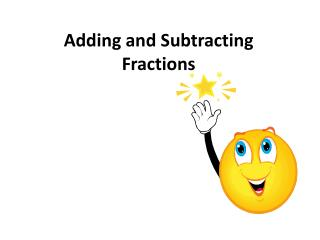DownloadDownload PresentationDownload Presentation- - - - - - - - - - - - - - - - - - - - - - - - - - - E N D - - - - - - - - - - - - - - - - - - - - - - - - - - -
##### Presentation Transcript

2. Let’s look at a model of adding fractions. Example: First we need a fraction bar representation of each addend. We will stack these so that the shading of the second starts where the shading of the first ends. To find the answer we need to change the second to the same number of sections that the first has. 3 sections out of whole Find the total of the shaded area.

3. Example Find • Remember: • Find fraction bars to represent both addends with the same number of sections • Stack these • Read answer

4. Let’s look at subtraction… Example We are going to look at a take away model. First build only the first term. Exchange these bars for an equivalent model using eighths. Now we need to imagine taking 5/8 away and find what will be left.

5. Our models show us that we can only add or subtract like terms. Rule: Whenadding orsubtracting fractions remember to rewrite the fractions with common denominators.

6. Once your fractions have a common denominator you will add or subtract the numerator and keep the denominator the same. Try…

7. Example #1

8. Example #2

9. Example #3

10. Example #4

11. Example #1 Vincent had ¼ of a yard of burlap to wrap his rose bushes before winter. He will need a total of ⅞ yard to wrap all of his roses. How much burlap should he purchase? Vincent needs to purchase ⅝ yard of burlap.